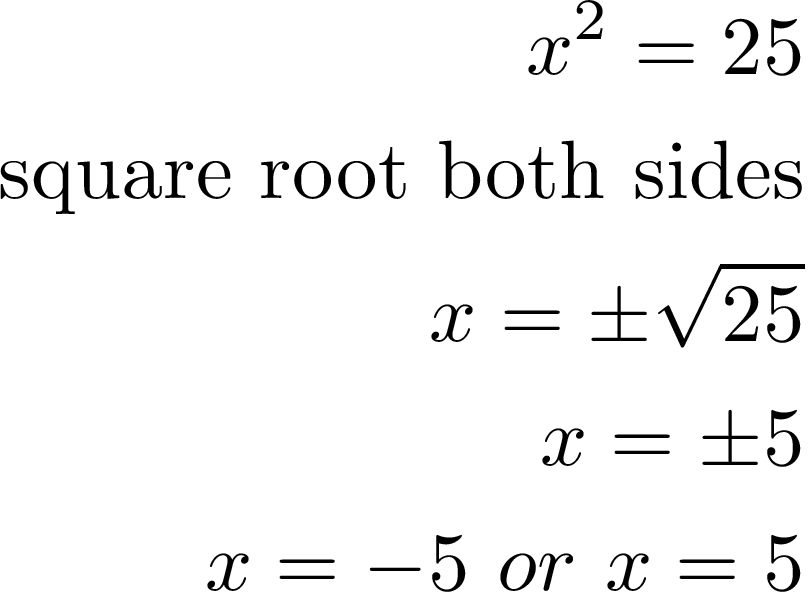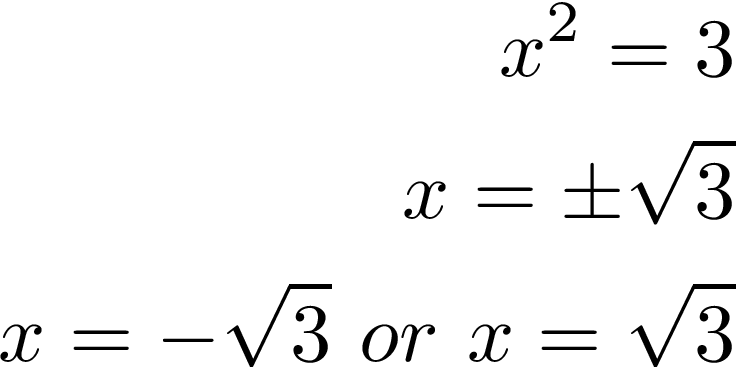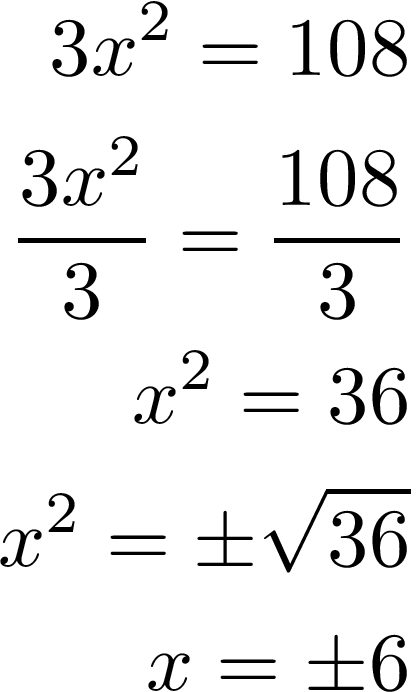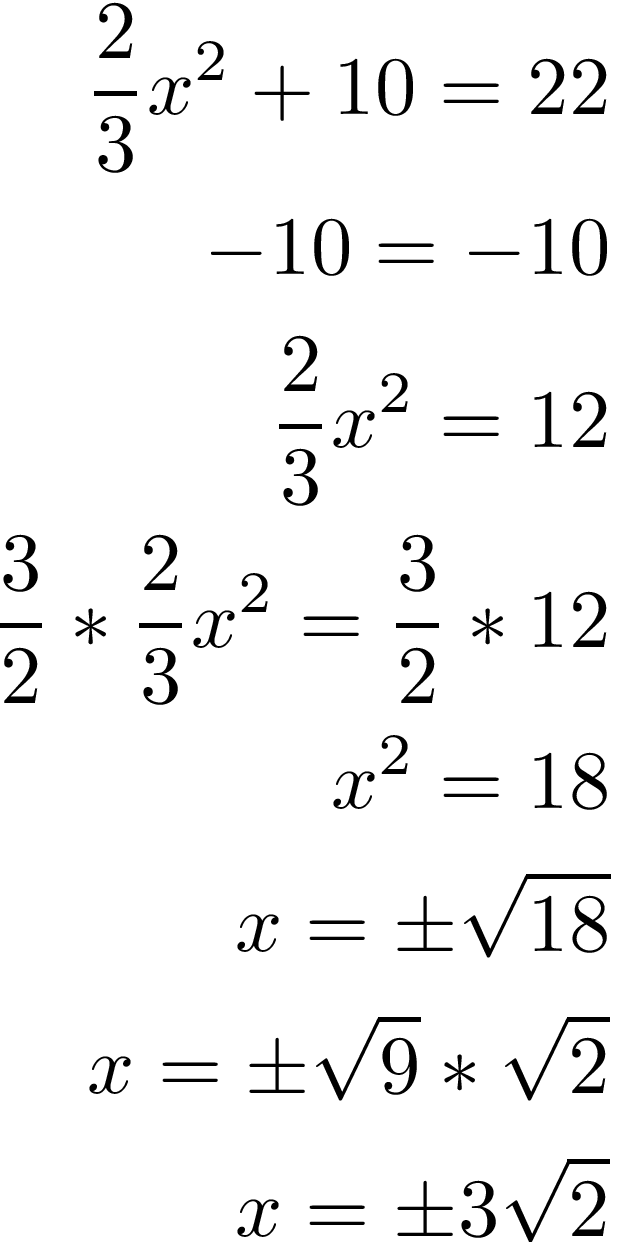# Quadratic Equations and the Square Root PropertyA quadratic equation is an equation that includes a variable raised to the second power. Below is a common format for a quadratic equation.This characteristic makes it difficult to rely on linear equation tricks of addition, subtraction, and multiplying to isolate the variable. One trick that we often have to use now is factoring as shown below.An alternative way to solve quadratic functions is through having knowledge of the square root property which is shown below.Below is the same example as our first example but this time we use the square root property.This trick works for numbers that cannot be factored.This leads us to the point that the square root property is used for speed or when factoring is not an option.

With this knowledge, all the other possible ways to solve a linear equation can be used to solve a quadratic equation

Division

In the example below is a quadratic formula in which you have to divide to isolate the variable. From there you solve like alwaysFraction

To remove a fraction you must multiply both sides by the reciprocal as shown below.When we got the square root of 18 we had to further simplify the radical by finding the factors of 18. In the second to last line if you multiply these numbers together you will get 18 because  9 * 2 = 18. Furthermore, if you square root 9 you get 3 but you cannot square root 2 and get a whole number. This is why the final answer is 3 * the square root of 2.

Conclusion

Quadratic formulas are common in algebra and as such there are many different ways to solve them. In this post, we looked at an alternative to factoring called the square root property. Understanding this approach is valuable as you can often solve quadratic equations faster and or they can be used when factoring is not possible.